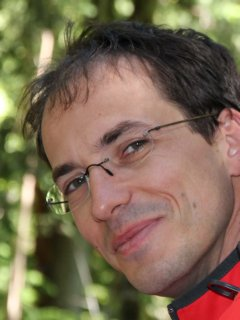# Prof.dr. Jan Bouwe van den Berg (VU Amsterdam)

Date:  07 March 2018

Time: 12:45 - 13:30 (Lunch available from 12:35)

Room: RA 1501 (Ravelijn)

Speaker: Prof.dr. Jan Bouwe van den Berg## Title: Computer-assisted theorems in dynamics

Abstract:
In nonlinear analysis we often simulate dynamics on a computer, or calculate a numerical solution to a partial differential equation. This gives very detailed, stimulating information. However, it would be even better if we can be sure that what we see on the screen genuinely represents a solution of the problem.
In particular, rigorous validation of the computations would allow such objects to be used as ingredients of theorems. In this talk we explore an approach based on a Newton-Kantorovich type argument in a suitable neighborhood of a numerically computed candidate. This method has been applied successfully for various problems in ordinary differential equations, delay differential equations and partial differential equations.
We will illustrate the general setup using an example stemming from the Navier-Stokes equations in two dimensions.

The latter is joint work in progress with Maxime Breden, Jean-Philippe Lessard and Lennaert van Veen.